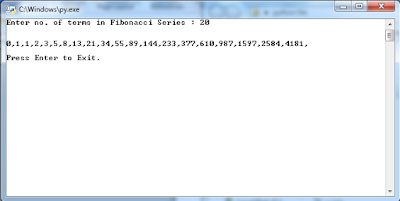## Tuesday, 29 May 2018

### To demonstrate use of slicing in string using python programming.

``` #Slicing in String print("SI = Starting index; EI = Ending index") nrStr = "I am Narendra Rajpoot." print("Length of Streing : ",len(nrStr)) print("String : ",nrStr) print("Charcter at index 3 : ",nrStr) print("String [SI = 3; EI = end of the sting] : ",nrStr[3:]) print("String [SI = 3; EI = 13] : ",nrStr[3:13]) print("String [SI = 0; EI = 10] ",nrStr[:10]) print("String [SI = 3; EI = 12; charcter differnce = 2]",nrStr[3:12:2]) input("\n\nPress Enter to exit.") ```

### To print ‘n terms of Fibonacci series using iteration using python programming.

``` n = int(input("Enter no. of terms in Fibonacci Series : ")) fTerm = 0 nTerm = 1 print("\n") for i in range(n):     if i<2:         print(i,end=",")     else:         temp = nTerm         nTerm = fTerm + nTerm         print(nTerm,end=",")         fTerm = temp input("\n\nPress Enter to Exit.") ```### To find all prime numbers within a given range using python programming.

``` # To find all prime numbers within a given range. import math n1 = int(input("Enter lower limit of range : ")) n2 = int(input("Enter upper limit of range : ")) print("\n") if n1==0:     n1+=1 if n1>0:     for i in range(n1,n2):         flag=0         sqr = math.sqrt(i)         for j in range(2,int(sqr)+1):             if j!=0:                 if (i%j)==0.0:                     flag=1                     break         if flag==0:             print(i,end=",") else:     print("Pleae enter positive limit: ") input("\n\nPress Any Key to Closse.") ```## Featured post

### In all other cases the driver is not insured. If the marital status, sex and age of the driver are the inputs, write a program to determine whether the driver is to be insured or not.

#include #include int main() { char ms;   printf("Is Driver married (Y/N): ");  scanf("%c",&ms);   if(ms=='y&#...